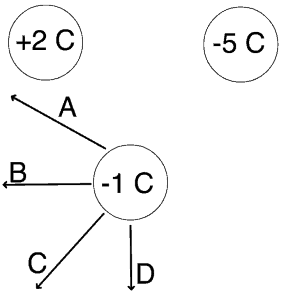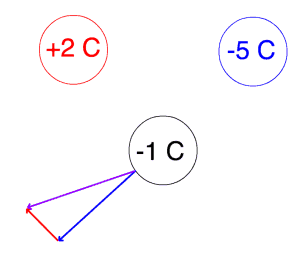# Free MCAT Practice Question, Round 3 – Physics

• by
• Jun 17, 2013
• MCAT Blog, MCAT Info, MCAT Long Form, MCAT Physics, MCAT Prep

# MCAT Physics Question Sample

Electrostatics and Magnetism is one of the classic parts of MCAT physics that gives students real trouble. As with all MCAT topics, the key lies in first mastering general principles before becoming bogged down in trying to memorize a ton of equations or learn nitpicky details. Here’s an example of question that tests one key principle about electrostatic force:

Item 14

The three ions in the figure below are held stationary in an equilateral triangle configuration.When the -1 C is released, which vector illustrates the direction the charge will initially move?

A) Vector A

B) Vector B

C) Vector C

D) Vector D

Explanation

This question tests two basic ideas: electrostatic force and vector addition. First we’ve got to remember that like charges repel and unlike charges attract. That means when the -1 C charge is released, it’s going to be pushed downwards by the other negative charge and pulled to the left by the positive charge:Only answer choice (C) correctly shows this net effect of being pushed down and to the left. Note that the force vector from the -5C charge is larger because it’s a larger charge, and Coulomb’s law tells us that the electrostatic force between two charges is direction proportional to their charge.